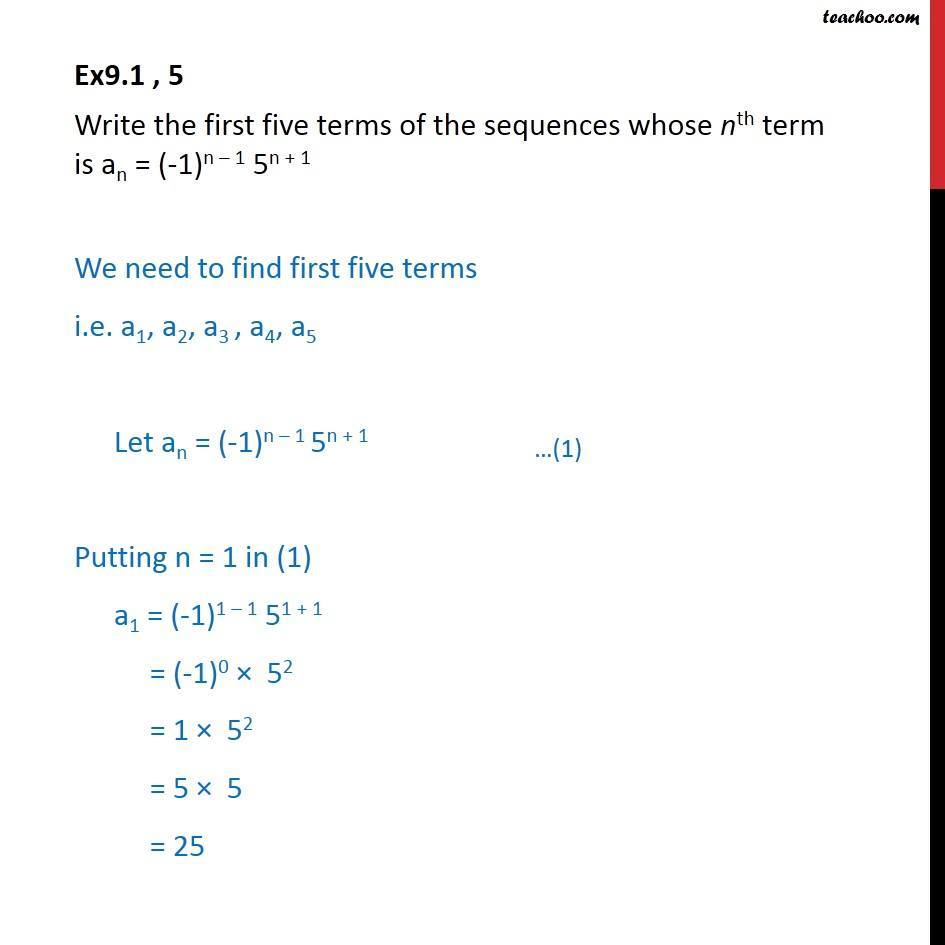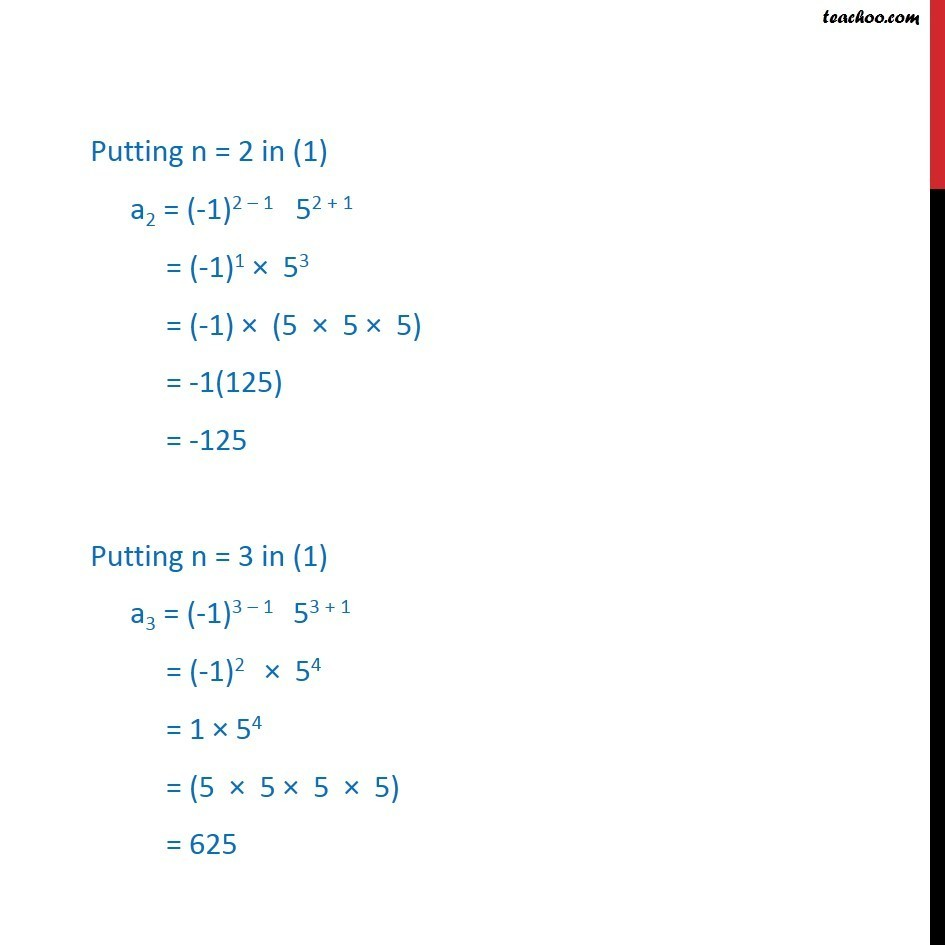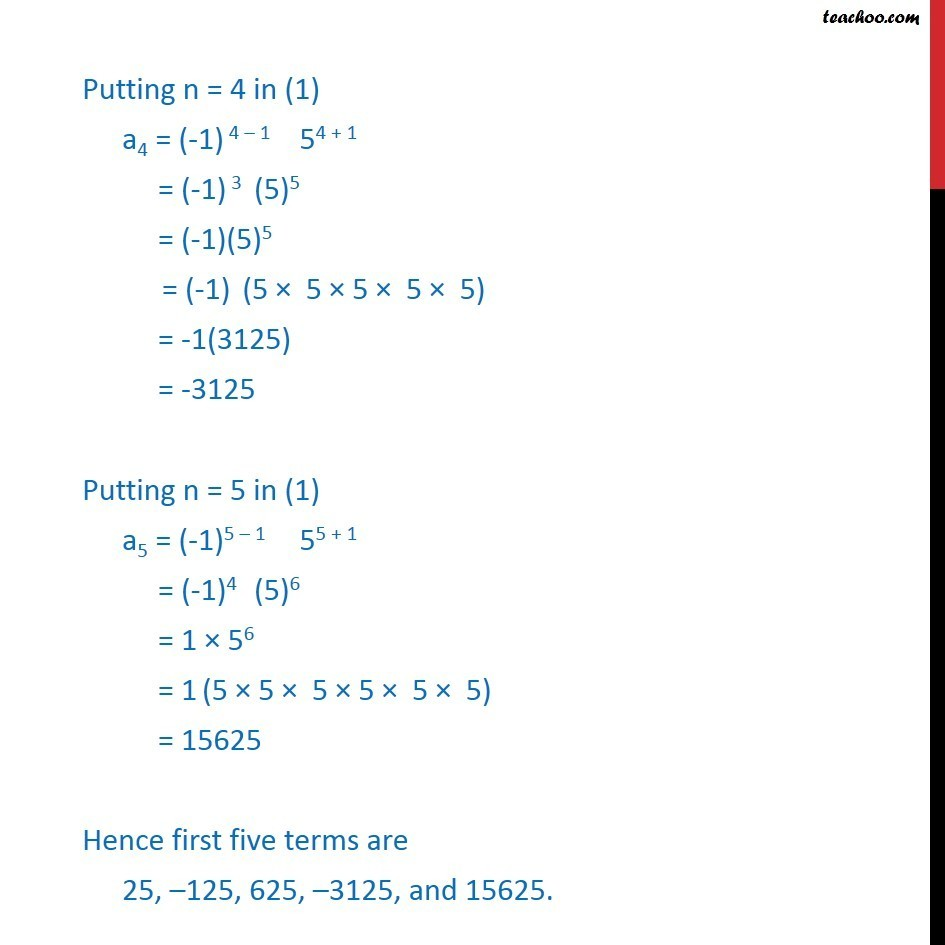Ex 8.1

Chapter 8 Class 11 Sequences and Series
Serial order wiseLearn in your speed, with individual attention - Teachoo Maths 1-on-1 Class

### Transcript

Ex9.1 , 5 Write the first five terms of the sequences whose nth term is an = (-1)n 1 5n + 1 We need to find first five terms i.e. a1, a2, a3 , a4, a5 Let an = (-1)n 1 5n + 1 Putting n = 1 in (1) a1 = (-1)1 1 51 + 1 = (-1)0 52 = 1 52 = 5 5 = 25 Putting n = 2 in (1) a2 = (-1)2 1 52 + 1 = (-1)1 53 = (-1) (5 5 5) = -1(125) = -125 Putting n = 3 in (1) a3 = (-1)3 1 53 + 1 = (-1)2 54 = 1 54 = (5 5 5 5) = 625 Putting n = 4 in (1) a4 = (-1) 4 1 54 + 1 = (-1) 3 (5)5 = (-1)(5)5 = (-1) (5 5 5 5 5) = -1(3125) = -3125 Putting n = 5 in (1) a5 = (-1)5 1 55 + 1 = (-1)4 (5)6 = 1 56 = 1 (5 5 5 5 5 5) = 15625 Hence first five terms are 25, 125, 625, 3125, and 15625.